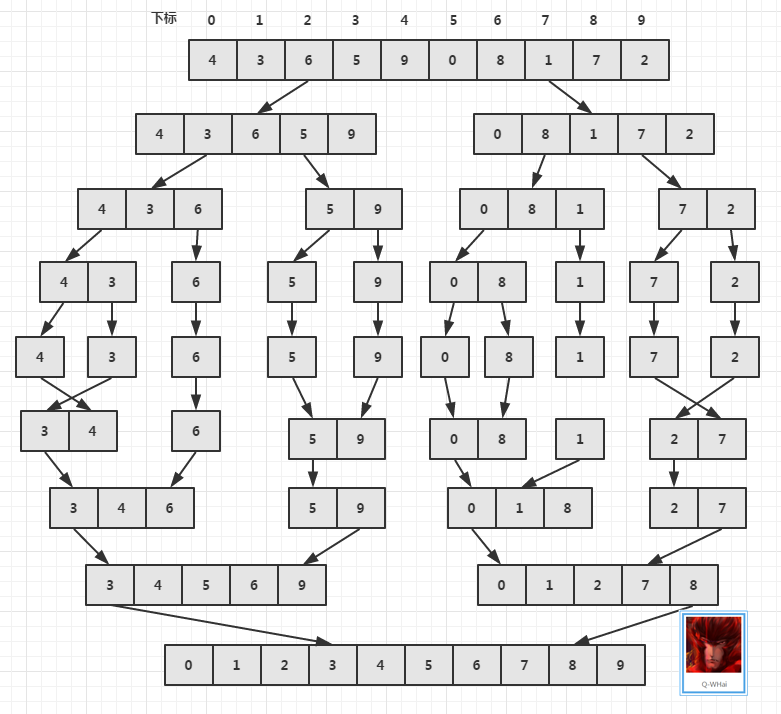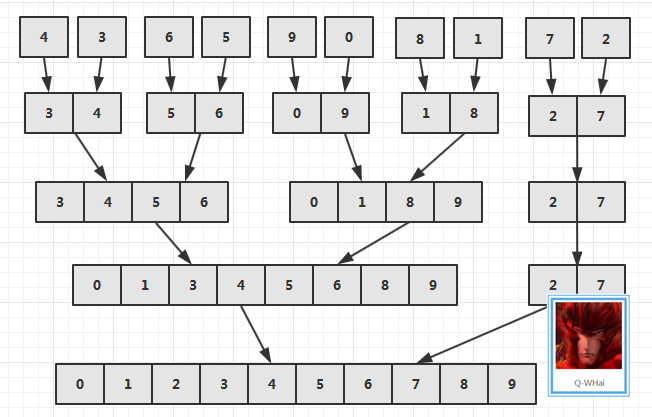• 递归算法相对来讲更好理解些，采用分治进行实现，在拆分的元素个数小于三个的时候进行排序。这里first，last和mid都为数组元素的下标值。 private void mergeSort1(int[] src, int temp[], int first, int last) {...
1.递归算法的实现
递归算法相对来讲更好理解些，采用分治法进行实现，在拆分的元素个数小于三个的时候进行排序。这里first，last和mid都为数组元素的下标值。

private void mergeSort1(int[] src, int temp[], int first, int last) {
if (last - first < 3) {// 拆分的元素个数小于等于3的时候进行排序
sort(src, first, last);
return;
}

int mid = (first + last) / 2;
mergeSort1(src, temp, first, mid);
mergeSort1(src, temp, mid + 1, last);

int i = first;// 二分归并第一组元素的头指针
int j = mid + 1;// 二分归并第二组元素的头指针
int k = first;// 临时数组的头指针

while (i <= mid && j <= last) {
temp[k++] = src[i] < src[j] ? src[i++] : src[j++];
}
while (i > mid && j <= last) {
</span>temp[k++] = src[j++];
}
while (j > last && i <= mid) {
temp[k++] = src[i++];
}

arrayCopy(src, temp, first, last);

}

// 将临时数组的数据拷贝到原数组当中temp->src
private void arrayCopy(int[] to, int[] from, int first, int last) {
for (int i = first; i <= last; ++i) {
to[i] = from[i];
}
}

private void arrayCopy(int[] src, int[] temp) {
arrayCopy(src, temp, 0, src.length - 1);
}

private void sort(int[] src, int first, int last) {
// 采用冒泡排序的方式对小于等于3个的拆分过的数组进行排序
int temp = 0;
for (int i = first; i < last; ++i) {
for (int j = first; j < last - i + first; ++j) {
if (src[j] > src[j + 1]) {
</span>temp = src[j];
</span>src[j] = src[j + 1];
</span>src[j + 1] = temp;
}
}
}
}

2.归并排序的迭代算法
归并排序的算法逻辑比较不容易理清，请大家自己代入几个数试一下很快就会明白。

private void mergeSort2(int[] src, int[] temp) {

//设置最开始分隔元素的距离为2

//		如果我们要排序的数组为5,4,3,2,1  那么第一次就应该对间隔为2的元素进行先简单排序再归并
//		排序后数组为4,5   2,3    1
//		然后此时对4,5   2,3  进行归并，1自然落下
//		而后数组变为2,3,4,5  然后space进行加倍，重复步骤，此时space不为2，所以不再进行排序，直接
//		进行归并

int space = 2;
// 下次是否是归并到原数组中的判断。  最开始的归并是从src到temp数组中进行的
boolean toSrc = false;
int len = src.length;

if(len == 2){
int temp1 = 0;
if(src>src){
temp1 = src;
src = src;
src = temp1;
}
}
int first = 0, last = 0, mid = 0;
while (space < len) {
for (int i = 0; i < len; i = last + 1) {
first = i;
mid = first + space - 1;
last = first + space * 2 - 1;
//在最末尾的元素大于数组最后一个元素的下标值时，将last设置为最后元素的下标值
if (last >= len - 1)
last = len - 1;
// 在mid<=last的时候 我们认为数组中最后剩余的元素可以进行归并而不是直接落入下次归并中
if (mid <= last) {
if (space == 2) {
compareForSwap(src, first, mid, last);
}
if (!toSrc) {
//向temp中进行归并
merge(src, temp, first, mid, last);
} else {
//向src中进行归并
merge(temp, src, first, mid, last);
}
}else{
if (!toSrc) {
//向temp中进行拷贝剩余元素
arrayCopy(temp, src, first, last);
} else {
//向src中进行拷贝剩余元素
arrayCopy(src, temp, first, last);
}

}

}
// 代表下一步该归并到那个数组中
toSrc = !toSrc;

space *= 2;
}
// 如果下一步该要归并到src中，代表现在已经正确归并的值留在了temp中 我们需要将他们复制到src中
if (toSrc) {
arrayCopy(src, temp);
}

}

//实现元素之间的比较判断以及交换
private void compareForSwap(int[] src, int first, int mid, int last) {
int temp = 0;
if (src[first] > src[mid]) {
temp = src[first];
src[first] = src[mid];
src[mid] = temp;
}
//这里用if判断使当最后的元素不足时不进行交换
if (mid + 1 < last) {
if (src[mid + 1] > src[last]) {
temp = src[mid + 1];
src[mid + 1] = src[last];
src[last] = temp;
}
}
}

private void merge(int[] from, int[] to, int first, int mid, int last) {
int i = first;// 二分归并第一组元素的头指针
int j = mid + 1;// 二分归并第二组元素的头指针
int k = first;// to数组的头指针

while (i <= mid && j <= last) {
to[k++] = from[i] < from[j] ? from[i++] : from[j++];
}
while (i > mid && j <= last) {
to[k++] = from[j++];
}
while (j > last && i <= mid) {
to[k++] = from[i++];
}
}

展开全文归并排序 归并排序递归 merge
• 归并排序（merge sort）算法的思想是
     归并排序（merge sort）算法的思想是（以数组为例）将一个要排序的数组分为两半，对这两半分别排序，再将它们归并为一个有序数组。这是一种采用分治法的算法，就是将一个问题分解成两个或更多个规模更小但却截然不同的问题，分别解决每个新问题，再将它们的解法组合起来解决原问题。综上所述，归并排序主要分为两个步骤：一是如何将要排序的数组进行划分;二是如何已经排好序的数组合并为原来的数组。下面是基于递归与迭代的归并排序算法的设计。
基于递归的merge sort
基本思路：将一个要排序的数组，按序号分成原数组的两半（为了合并的方便，要保证划分后的数组序号是连续的），对这两半分别排序，将排序后的两个数组合并为一个临时数组，最后将这个临时数组复制回原数组。（注：划分后的两个数组继续使用划分的方法进行划分，只到每个数组中只含一个元素为止）
算法实现：
/**
* 这一步主要是为了隐藏临时数组
* @param Array
* @param first
* @param last
*/
public void mergeSort(T[] Array,int first,int last)
{
T[] tempArray=(T[])new Comparable<?>[Array.length];
mergeSort(Array,tempArray,first,last);
}
/**
* 归并排序
* @param Array  原数组
* @param tempArray  临时数组
* @param first  要排序数组的起始序号
* @param last   要排序数组的结束序号
*/
public void mergeSort(T[] Array,T[] tempArray,int first,int last)
{

if(first<last)
{
int mid=(first+last)/2;
mergeSort(Array,tempArray,first,mid);
mergeSort(Array,tempArray,mid+1,last);
merge(Array,tempArray,first,mid,last);
}
}
/**
* 将已经排好序的数组通过临时数组合并成原来的数组
* @param Array   原数组
* @param tempArray  临时数组
* @param first   要排序数组的起始序号
* @param mid     将数组分割成两半的序号
* @param last    要排序数组的结束序号
*/
public void merge(T[] Array,T[] tempArray,int first,int mid,int last)
{
int leftIndex=first;
int rightIndex=mid+1;
int i=first;
while(leftIndex<=mid&&rightIndex<=last)
{
if(Array[leftIndex].compareTo(Array[rightIndex])<0)
{
tempArray[i]=Array[leftIndex];
leftIndex++;
}
else
{
tempArray[i]=Array[rightIndex];
rightIndex++;
}
i++;
}
// 将左半边剩余的数组复制到临时数组中
while(leftIndex<=mid)
{
tempArray[i++]=Array[leftIndex++];
}
// 将右半边剩余的数组复制到临时数组中
while(rightIndex<=last)
{
tempArray[i++]=Array[rightIndex++];
}
// 将临时数组复制回原数组
for(int j=first;j<=last;j++)
{
Array[j]=tempArray[j];
}
}       基于迭代的merge sort

基本思路：从数组的始端开始，将每一对单个元素归并为含有两个元素的子数组，然后回到数组的始端，将每一对含有两个元素的子数组归并为含有4个元素的子数组，以此类推。注：归并两个子数组需要一个额外的临时数组，尽管使用这额外的数组的空间是必需的，但可以通过一定的技巧节省递归中消耗在将元素从临时数组复制回原数组的大部分时间。
算法实现：

	/**
* 将Array中的子数组归并到tempArray中，不需要将tempArray复制到Array中，而是将tempArray的子数组归并到Array中
* @param Array
* @param first
* @param last
*/
public void mergetionSort(T[] Array,int first,int last)
{
T[] tempArray=(T[])new Comparable<?>[Array.length];
int step=1;
while(step<last)
{
mergePass(Array,tempArray,step,first,last);
for(int i=0;i<tempArray.length;i++)
System.out.print(tempArray[i]+" ");
System.out.println();
step*=2;
mergePass(tempArray,Array,step,first,last);
for(int i=0;i<Array.length;i++)
System.out.print(Array[i]+" ");
System.out.println();
step*=2;
}
}

/**
* 对Array表按子表步长为step, [0,step-1],[step,step*2-1],归并到MergeList中
* @param Array   原数组
* @param tempArray 临时数组
* @param step  步长从1开始，然后按2的倍数进行递增（即指子数组中的元素）
*/
public void mergePass(T[] Array,T[] tempArray,int step,int first,int last)
{
int index=first;//下一个待归并段开始指针
while(index<=last)
{
//剩余元素存在两个待归并长度的元素
if(index+2*step<=(last+1))
{
merge2(Array,tempArray,index,index+step-1,index+step*2-1);
index=index+2*step;  //将指针进行迭代
}
//剩余元素存在一个待归并长度的元素和一个小于其归并长度的元素
else if(index+step<=(last+1))
{
merge2(Array,tempArray,index,index+step-1,last);
index=last+1;
}
//存在一个小于其归并长度的元素
else
while(index<=last)
{
tempArray[index]=Array[index];
index++;
}
}
}
/**
* 将已经排好序的数组通过临时数组合并成原来的数组
* @param Array   原数组
* @param tempArray  临时数组
* @param first   要排序数组的起始序号
* @param mid     将数组分割成两半的序号
* @param last    要排序数组的结束序号
*/
public void merge2(T[] Array,T[] tempArray,int first,int mid,int last)
{
int leftIndex=first;
int rightIndex=mid+1;
int i=first;
while(leftIndex<=mid&&rightIndex<=last)
{
if(Array[leftIndex].compareTo(Array[rightIndex])<0)
{
tempArray[i]=Array[leftIndex];
leftIndex++;
}
else
{
tempArray[i]=Array[rightIndex];
rightIndex++;
}
i++;
}
// 将左半边剩余的数组复制到临时数组中
while(leftIndex<=mid)
{
tempArray[i++]=Array[leftIndex++];
}
// 将右半边剩余的数组复制到临时数组中
while(rightIndex<=last)
{
tempArray[i++]=Array[rightIndex++];
}
}


展开全文递归 merge
• 面试 11：Java 玩转归并排序 前面讲了冒泡、选择、插入三种简单排序，时间复杂度都是 O(n²)，今天，我们终于迎来了更高级的排序：归并排序。 虽然在这之前还有希尔排序和堆排序，但由于时间关系，我们这里就直接跳...


面试 11：Java 玩转归并排序
前面讲了冒泡、选择、插入三种简单排序，时间复杂度都是 O(n²)，今天，我们终于迎来了更高级的排序：归并排序。
归并排序
我们总是可以将一个数组一分为二，然后二分为四，直到每一组只有两个元素，这可以理解为个递归的过程，然后将两个元素进行排序，之后再将两个元素为一组进行排序。直到所有的元素都排序完成。同样我们来看下边这个动图。

图片来源于网络

归并排序算法是采用分治法的一个非常典型的应用，且各层分治递归可以同时进行。
归并算法的思想
归并算法其实可以分为递归法和迭代法（自底向上归并），两种实现对于最小集合的归并操作思想是一样的。区别在于如何划分数组，我们先介绍下算法最基本的操作：
申请空间，使其大小为两个已经排序序列之和，该空间用来存放合并后的序列；
设定两个指针，最初位置分别为两个已经排序序列的起始位置；
比较两个指针所指向的元素，选择相对小的元素放入到合并空间，并移动指针到下一位置；
重复步骤 3 直到某一指针到达序列尾；
将另一序列剩下的所有元素直接复制到合并序列尾。
我们来看看 Java 递归代码是怎么实现的：
public class Test09 {

private static void swap(int[] arr, int i, int j) {
int temp = arr[i];
arr[i] = arr[j];
arr[j] = temp;
}

private static void printArr(int[] arr) {
for (int anArr : arr) {
System.out.print(anArr + " ");
}
}

private static void mergeSort(int[] arr) {
if (arr == null)
return;
mergeSort(arr, 0, arr.length - 1);
}

private static void mergeSort(int[] arr, int start, int end) {
if (start >= end)
return;
// 找出中间索引
int mid = start + (end - start >> 1);
// 对左边数组进行递归
mergeSort(arr, start, mid);
// 对右边数组进行递归
mergeSort(arr, mid + 1, end);
// 合并
merge(arr, start, mid, end);
}

private static void merge(int[] arr, int start, int mid, int end) {
// 先建立一个临时数组，用于存放排序后的数据
int[] tmpArr = new int[arr.length];

int start1 = start, end1 = mid, start2 = mid + 1, end2 = end;
// 创建一个下标
int pos = start1;
// 缓存左边数组的第一个元素的索引
int tmp = start1;
while (start1 <= end1 && start2 <= end2) {
// 从两个数组中取出最小的放入临时数组
if (arr[start1] <= arr[start2])
tmpArr[pos++] = arr[start1++];
else
tmpArr[pos++] = arr[start2++];
}
// 剩余部分依次放入临时数组，实际上下面两个 while 只会执行其中一个
while (start1 <= end1) {
tmpArr[pos++] = arr[start1++];
}
while (start2 <= end2) {
tmpArr[pos++] = arr[start2++];
}
// 将临时数组中的内容拷贝回原来的数组中
while (tmp <= end) {
arr[tmp] = tmpArr[tmp++];
}

}

public static void main(String[] args) {
int[] arr = {6, 4, 2, 1, 8, 3, 7, 9, 5};
mergeSort(arr);
printArr(arr);
}
}

归并排序算法总的时间复杂度是 O(nlogn)，而且这是归并排序算法中最好、最坏、平均的时间性能。
而由于在归并排序过程中需要与原始记录序列同样数量的存储空间存放归并结果以及递归时压入栈的数据占用的空间：n + logn，所以空间复杂度为 O(n)。
总结
归并排序虽然比较稳定，在时间上也是非常有效的，但是这种算法很消耗空间，一般来说只有在外部排序才会采用这个方法，但在内部排序不会用这种方法，而是用快速排序。明天，我们将带来排序算法中的王牌：快速排序。


展开全文• 归并排序迭代方法的原理及实现
1. 基本原理

在上篇文章中介绍了归并排序的递归实现，虽然递归的实现方式很简单，通过递归调用就可以实现，但是会占用大量的时间和空间，使得算法的效率下降；使用迭代的方式代替递归的方式虽然会使得代码的编写变得困难，但是会增大效率。

递归的思想实际上是从上往下“递”，再从下往上“归”。递归的实现过程可以看成如下的过程而迭代的过程就是化成一个个小的排序问题，再合并到一起，比如如下的过程2. 代码实现

#include <stdio.h>
#include <stdlib.h>

#define MAXSIZE 10

void MergeSort(int k[], int n)
{
// i 用于 for 循环迭代,temp 用来存储临时数组，所以要给他分配内存
int i, next, left_min, left_max, right_min, right_max;
int *temp = (int *)malloc(n * sizeof(int));

// i 是步长，第一次是1个元素与1个元素相比，第2个是两个元素与2个元素相比
for( i=1; i < n; i*=2 )
{
// left_min 最后是要小于 n-i 的，这一点可以通过画图得到
// left_min = right_max 是指上一组排序完成之后，将上一组的 right 赋值给下一组的 left_min
for( left_min=0; left_min < n-i; left_min = right_max )
{
right_min = left_max = left_min + i;
right_max = left_max + i;

// 因为奇数的数组最后很有可能 right_max > n，所以将它限制到最大为 n
if( right_max > n )
{
right_max = n;
}

next = 0;

// 在这里跟递归中的归并操是一样的，从两个数组中取小的出来放入临时数组
while( left_min < left_max && right_min < right_max )
{
if( k[left_min] < k[right_min] )
{
temp[next++] = k[left_min++];
}
else
{
temp[next++] = k[right_min++];
}
}

// 但是上面的过程并不会同时将左右两个数组的元素都放入临时存储数组 temp 中
// 要么左边会剩一点，要么右边会剩一点，所以这个时候要对剩余的进行操作
// 如果左边剩了，说明这些应该是最大的，应该放在数组的右边
while( left_min < left_max )
{
k[--right_min] = k[--left_max];
}

// 将临时存储的元素放入数组中得到最后的结果
while( next > 0 )
{
k[--right_min] = temp[--next];
}
}
}
}

int main()
{
int i, a = {5, 2, 6, 0, 3, 9, 1, 7, 4, 8};

MergeSort(a, 10);

printf("排序后的结果是：");
for( i=0; i < 10; i++ )
{
printf("%d", a[i]);
}
printf("\n\n");

return 0;
}

参考文献

Q-WHai ，排序算法系列：归并排序算法
展开全文排序算法 数据结构与算法
• 归并排序是建立在归并操作上的一种有效的排序算法。该算法是采用分治的一个非常典型的应用。归并排序的实现有两种方法：（1）自上而下的递归；（2）自下而上的迭代。 算法步骤 （1）申请空间，使其大小为两个已经...
• 归并排序（Meger-Sort）是利用归并的思想实现的排序方法，该算法采用经典的分治（divide-and-conquer）策略（分治将问题分成一些小的问题，然后递归求解，而治的阶段则将分的阶段得到的各个答案“修补”在一起，即...算法 后端
• 归并排序是建立在归并操作上的一种有效的排序算法，1945年由约翰·冯·诺伊曼首次提出。该算法是采用分治（Divide and Conquer）的一个非常典型的应用，且各层分治递归可以同时进行。 1、基本思想 归并排序算法...
• 归并排序(MERGE-SORT)是利用归并的思想实现的排序方法，该算法采用经典的分治(divide-and-conquer) 策略(分治将问题分(divide)成一些小的问题然后递归求解，而治(conquer)的阶段则将分的阶段得到的各答案"修 补"在...
• 八大排序算法 一、直接插入 - 1.基本思路 - 2.代码实现 - 3.时间复杂度和空间复杂度 二、希尔排序 - 1.基本思路 - 2.代码实现 - 3.时间复杂度和空间复杂度 三、简单选择 - 1.基本思路 - 2.代码实现 - 3.时间复杂度...算法 数据结构
• 归并排序建立在归并操作上的一种有效，稳定的排序算法，是采用分治的一个非常典型的应用。将集合数据拆分成若干个子序列，并使各个子序列有序，依次合并，并进行排序，直到合并为整个数据集合有序。（此处应该有...算法 数据结构
• 归并排序（MERGE-SORT）是利用归并的思想实现的排序方法，该算法采用经典的分治（divide-and-conquer）策略（分治将问题分(divide)成一些小的问题然后递归求解，而治(conquer)的阶段则将分的阶段得到的各答案"修补...
• 归并排序 我们总是可以将一个数组一分为二，然后二分为四，直到每一组只有两个元素，这可以理解为个递归的过程...归并算法其实可以分为递归法和迭代法（自底向上归并），两种实现对于最小集合的归并操作思想是一样...
• 归并排序是利用归并的思想实现的排序算法，该算法采用经典的分治策略(分治是将问题分成一些小的问题然后递归求解，而治的阶段则将分的阶段得到的各答案"修补"在一起，即分而治之)。 基本思想： 分的阶段就是递归拆...
• 归并排序从小到大排序 我们总是可以将一个数组一分为二，然后二分为四，直到每一组只有两个元素，这可以理解为个递归的过程，然后将两个元素进行排序，之后再将两个元素为一组进行排序。直到所有的元素都排序完成。 ...
• 合并排序技术使用“分而治之”策略。在这种技术中，将要排序的数据集被划分为较小的单元以对其进行排序...在本教程中，我们将全面讨论该排序技术的所有细节，包括其算法和伪代码以及该技术在Java中的实现。 Java中的M.
• —— 约翰·冯·诺伊曼归并排序简介归并排序(Merge Sort)，是创建在归并操作上的一种有效的排序算法。1945年由约翰·冯·诺伊曼首次提出。该算法是采用分治(Divide and Conquer)的一个非常典型的应用，且各层分治...排序算法
• 　归并排序（MERGE-SORT）是利用归并的思想实现的排序方法，该算法采用经典的分治（divide-and-conquer）策略（分治将问题分(divide)成一些小的问题然后递归求解，而治(conquer)的阶段则将分的阶段得到的各答案...
• 归并排序（MERGE-SORT）是利用归并的思想实现的排序方法，该算法采用经典的分治（divide-and-conquer）策略（分治将问题分(divide)成一些小的问题然后递归求解，而治(conquer)的阶段则将分的阶段得到的各答案"修补...算法 数据结构
• 归并排序 归并排序（merge sort）是建立在归并操作上的一种有效的排序算法。该算法是采用分治的一个非常典型的应用。作为一种典型的分而治之思想的算法应用，归并排序的实现有两种方法： 自上而下的递归（所有...
• 归并排序（Merge sort），是分治（divide-and-conquer）的典型应用。其基本思想是将待排序序列划分成两个子序列，分别将两子序列排好序，再将这两子序列合并成一个有序序列，而两个子序列又可以应用这种归并策略。...排序算法 Merge C语言
• 归并排序是建立在归并操作上的一种有效的排序算法，采用分治（Divide and Conquer）思想，且各层分治递归可以同时进行。归并排序是稳定的排序算法。 基本思想 归并排序算法是将两个（或两个以上）有序表合并成一个...数据结构 算法
• 在介绍归并排序之前,先简单的说一下O(NlogN)和O(N2)之间的比较,通过下面的图片可以明显的看出来,前者的优势是很明显的,并且随着N的增大,优势会越来越明显,优化之后的代码可能意味着笨的算法一辈子都算不出来结果,而...算法 分治算法
• 　归并排序（MERGE-SORT）是利用归并的思想实现的排序方法，该算法采用经典的分治（divide-and-conquer）策略（分治将问题分(divide)成一些小的问题然后递归求解，而治(conquer)的阶段则将分的阶段得到的各答案&...
• 归并排序算法思想是建立在分治之上的，思路简单，时间复杂度不会过于复杂，主要适用于总体无序，但子序列相对有序的情况。 基本步骤       归并排序既可以采用递归思想，也可以采用迭代思想，无论...算法
• 图解排序算法(四)之归并排序基本思想　归并排序（MERGE-SORT）是利用归并的思想实现的排序方法，该算法采用经典的分治（divide-and-conquer）策略（分治将问题分(divide)成一些小的问题然后递归求解，而治(conquer...算法
• 3. 迭代归并排序的复杂度分析  关于插入排序的复杂度分析， 我们基于其伪代码实现来进行分析：    其运行的总时间为：   其在最坏情况下的总时间为：    总时间为 N*N的正相关关系， 故其消耗时间为：    ...算法分析 复杂度...

# java归并排序迭代法java 订阅